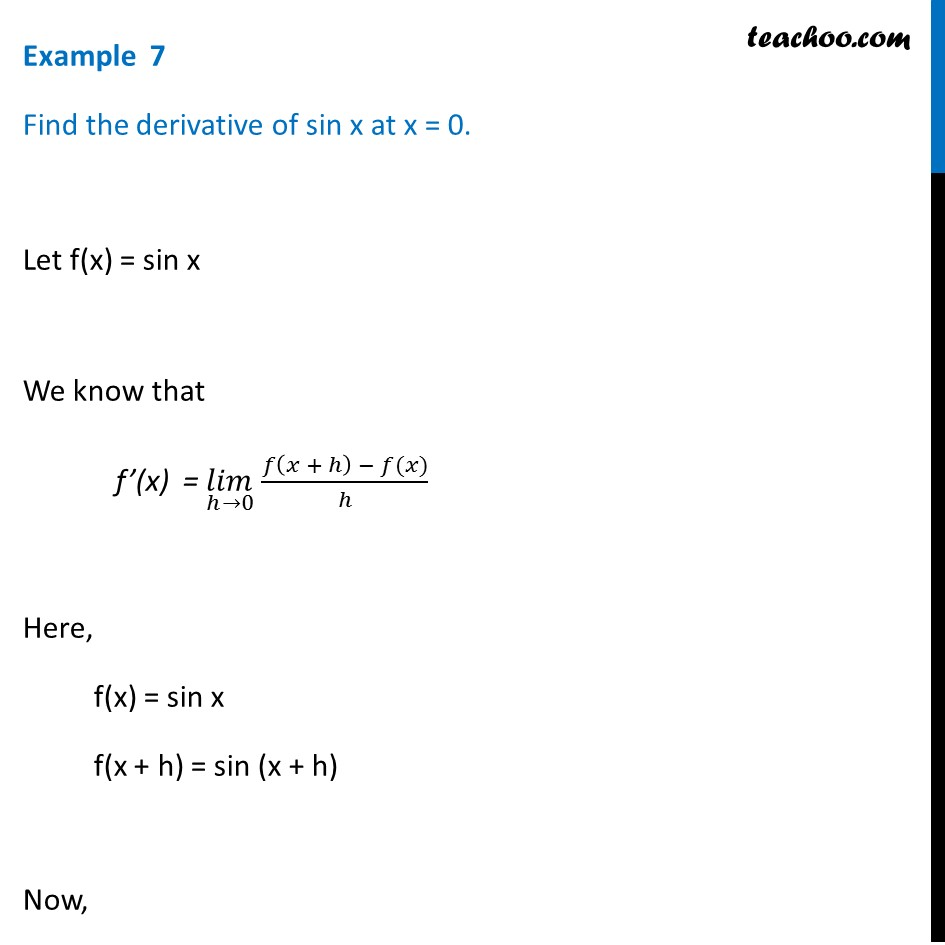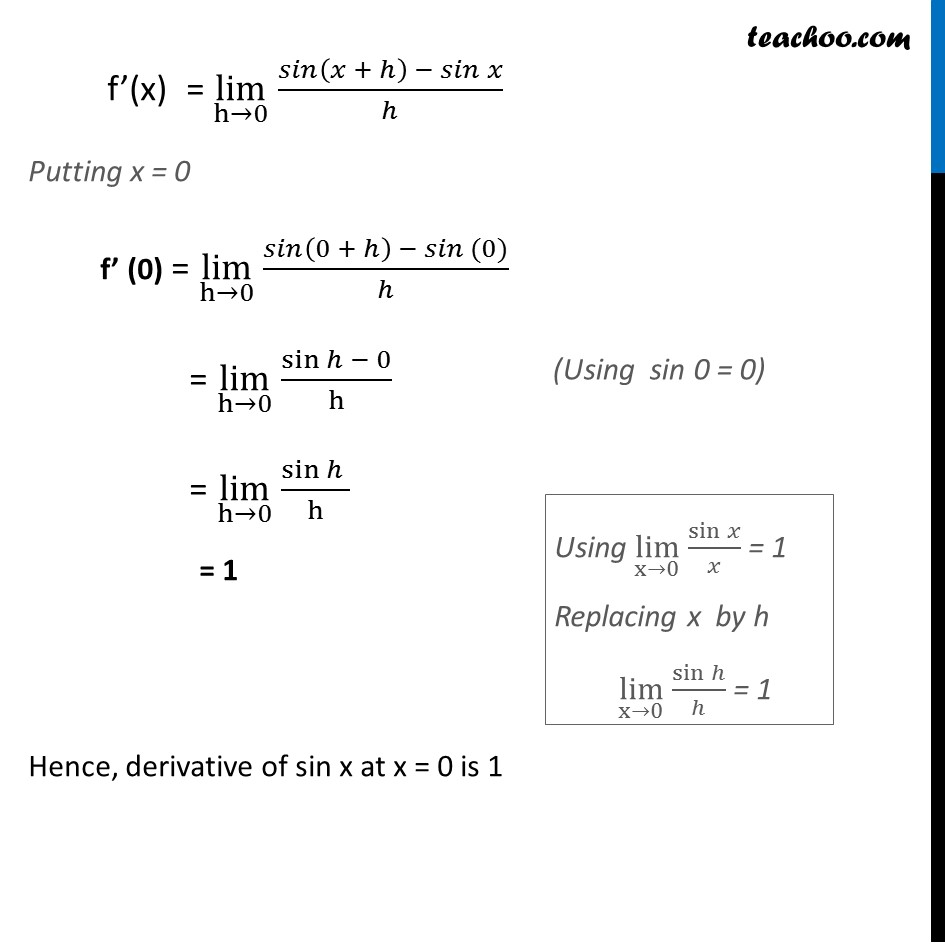1. Chapter 13 Class 11 Limits and Derivatives
2. Concept wise
3. Derivatives by 1st principle - At a point

Transcript

Example 7 Find the derivative of sin x at x = 0. Let f(x) = sin x We know that f’(x) = (𝑙𝑖𝑚)┬(ℎ→0) 𝑓⁡〖(𝑥 + ℎ) − 𝑓(𝑥)〗/ℎ Here, f(x) = sin x f(x + h) = sin (x + h) Now, f’(x) = lim┬(h→0) 𝑠𝑖𝑛⁡〖(𝑥 + ℎ) − 𝑠𝑖𝑛 𝑥〗/ℎ Putting x = 0 f’ (0) = lim┬(h→0) 𝑠𝑖𝑛⁡〖(0 + ℎ) − 𝑠𝑖𝑛 (0)〗/ℎ = lim┬(h→0) sin⁡〖ℎ − 0〗/h = lim┬(h→0) sin⁡〖ℎ 〗/h = 1 Hence, derivative of sin x at x = 0 is 1 Using lim┬(x→0) sin⁡𝑥/𝑥 = 1 Replacing x by h lim┬(x→0) sin⁡ℎ/ℎ = 1

Derivatives by 1st principle - At a point

About the AuthorDavneet Singh
Davneet Singh is a graduate from Indian Institute of Technology, Kanpur. He has been teaching from the past 10 years. He provides courses for Maths and Science at Teachoo.